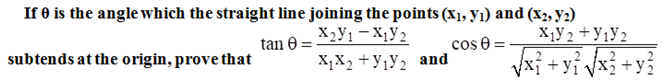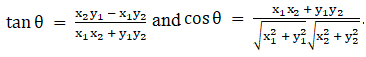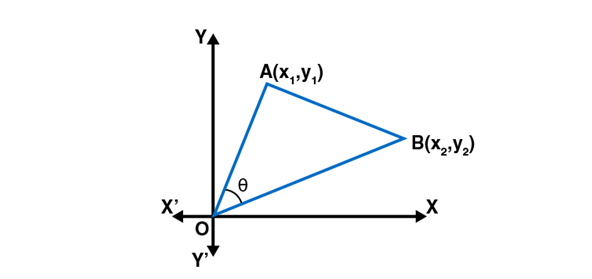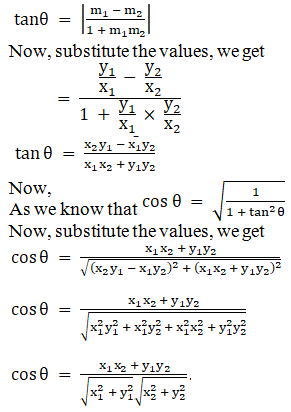• +91 9971497814
• info@interviewmaterial.com

# RD Chapter 23- The Straight Lines Ex-23.13 Interview Questions Answers

### Related Subjects

Question 1 :
Find the angles between each of the following pairs of straight lines:
(i) 3x + y + 12 = 0 and x + 2y – 1 = 0
(ii) 3x – y + 5 = 0 and x – 3y + 1 = 0

(i) 3x + y + 12 = 0 and x+ 2y – 1 = 0

Given:

The equations of thelines are

3x + y + 12 = 0 … (1)

x + 2y − 1 =0 … (2)

Let m1 andm2 be the slopes of these lines.

m1 =-3, m2 = -1/2

Let θ be theangle between the lines.

Then, by using theformula

tan θ = [(m1 –m2) / (1 + m1m2)]

= [(-3 + 1/2) / (1 +3/2)]

= 1

So,

θ = π/4 or 45o

The acute anglebetween the lines is 45°

(ii) 3x – y + 5 = 0 and x –3y + 1 = 0

Given:

The equations of thelines are

3x − y + 5 =0 … (1)

x − 3y + 1 =0 … (2)

Let m1 andm2 be the slopes of these lines.

m1 =3, m2 = 1/3

Let θ be theangle between the lines.

Then, by using theformula

tan θ = [(m1 –m2) / (1 + m1m2)]

= [(3 + 1/3) / (1 +1)]

= 4/3

So,

θ = tan-1 (4/3)

The acute anglebetween the lines is tan-1 (4/3).

Question 2 : Find the acute angle between the lines 2x – y + 3 = 0 and x + y + 2 = 0.

Given:

The equations of thelines are

2x − y + 3 =0 … (1)

x + y + 2 = 0 … (2)

Let m1 andm2 be the slopes of these lines.

m1 =2, m2 = -1

Let θ be theangle between the lines.

Then, by using theformula

tan θ = [(m1 –m2) / (1 + m1m2)]

= [(2 + 1) / (1 + 2)]

= 3

So,

θ = tan-1 (3)

The acute anglebetween the lines is tan-1 (3).

Question 3 : Prove that the points (2, -1), (0, 2), (2, 3) and (4, 0) are the coordinates of the vertices of a parallelogram and find the angle between its diagonals.

To prove:

The points (2, -1),(0, 2), (2, 3) and (4, 0) are the coordinates of the vertices of a parallelogram

Let us assume thepoints, A (2, − 1), B (0, 2), C (2, 3) and D (4, 0) be the vertices.

Now, let us find theslopes

Slope of AB = [(2+1) /(0-2)]

= -3/2

Slope of BC = [(3-2) /(2-0)]

= ½

Slope of CD = [(0-3) /(4-2)]

= -3/2

Slope of DA = [(-1-0)/ (2-4)]

= ½

Thus, AB is parallelto CD and BC is parallel to DA.

Hence proved, thegiven points are the vertices of a parallelogram.

Now, let us find theangle between the diagonals AC and BD.

Let m1 andm2 be the slopes of AC and BD, respectively.

m1 =[(3+1) / (2-2)]

= ∞

m2 =[(0-2) / (4-0)]

= -1/2

Thus, the diagonal ACis parallel to the y-axis.

ODB = tan-1 (1/2)

In triangle MND,

DMN = π/2 – tan-1 (1/2)

The angle between thediagonals is π/2 – tan-1 (1/2).

Question 4 : Find the angle between the line joining the points (2, 0), (0, 3) and the line x + y = 1.

Given:

Points (2, 0), (0, 3)and the line x + y = 1.

Let us assume A (2,0), B (0, 3) be the given points.

Now, let us find theslopes

Slope of AB = m1

= [(3-0) / (0-2)]

= -3/2

Slope of the line x +y = 1 is -1

m2 =-1

Let θ be theangle between the line joining the points (2, 0), (0, 3) and the line x + y =

tan θ = |[(m1 –m2) / (1 + m1m2)]|

= [(-3/2 + 1) / (1 +3/2)]

= 1/5

θ = tan-1 (1/5)

The acute anglebetween the line joining the points (2, 0), (0, 3) and the line x + y = 1is tan-1 (1/5).

Question 5 :We need to prove:Let us assume A (x1,y1) and B (x2, y2) be the given points and Obe the origin.

Slope of OA = m1 =y1×1

Slope of OB = m2 =y2×2

It is giventhat θ is the angle between lines OA and OB.Hence proved.

Todays Deals### RD Chapter 23- The Straight Lines Ex-23.13 Contributorskrishan

Name:
Email:

# Latest News# 9000 interview questions in different categories